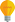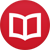# How to translate chord progressions into any scale easily

by Jakub MarianTip: See my list of theMost Common Mistakes in English. It will teach you how to avoid mis­takes with com­mas, pre­pos­i­tions, ir­reg­u­lar verbs, and much more.

In the previous article, we learned that it is possible to avoid memorization in music theory by using numbers instead of letters for note naming. We used the following table:

 … D♭ A♭ E♭ B♭ F C G D A E B F♯ C♯ G♯ D♯ … … -7 -6 -5 -4 -3 -2 -1 0 1 2 3 4 5 6 7 …

Now we will learn how to use this approach in practice. In the traditional Roman numeral harmony analysis, chord progressions look like I – IV – V – I. This notation is useful, because it’s an abstract notation which allows you to notate chord progressions without relation to any particular scale. However, if I tell you to write down the chord names in D♭ major, A major, and F♯ major, there are two possibilitieseither you have already memorized what IV and V mean in these scales, or you will have to basically recite the alphabet in your head starting from D, A, and F, respectively (to find the fourth and the fifth letter after D, A, F, with A being the next after G), and then try to recall whether these get an accidental or not.

Using numbers, this gets much easier. Chord progressions become just patterns of addition and subtraction. For example, “I – IV – V – I” is “$x$, $x-1$, $x+1$, $x$”, where $x$ is the scale we are in. For example, if we are in $2$ major (E major), it is $2$, $1$, $3$, $2$ (E, A, B, E), in $-2$ major (C major) it is $-2$, $-3$, $-1$, $-2$ (C, F, G, C), in $4$ major (F♯ major) it is $4$, $3$, $5$, $4$ (F♯, B, C♯, F♯)… And you would certainly be able to translate the progression into any other scale now.

The point is that any conceivable chord progression now becomes a simple pattern. Do you want to learn the most ubiquitous chord progression in contemporary music, I – vi – ii – V – I in all major scales? Once you remember that it is “three up, down, down, down” (e.g. $0$ $3$m $2$m $1$ $0$, where “m” means a minor chord), the process will be trivial when you use numbers; compare a few progressions written in the traditional way with our new way:

 Key Standard notation Key Numeral notation D D – Bm – Em – A – D 0 $0$ $3$m $2$m $1$ $0$ B B – G♯m – C♯m – F♯ – B 3 $3$ $6$m $5$m $4$ $3$ G♯ G♯ – E♯m – A♯m – D♯ – G♯ 6 $6$ $9$m $8$m $7$ $6$

Another important property of the numeral notation is that this process can be easily reversed. For example, if we are to analyse the progression

$4$ $3$ $5$ $4$ $7$m $6$m $5$ $4$

in the scale of $4$, we would simply subtract 4 from all the notes, which would lead to

$0$ $-1$ $1$ $0$ $3$m $2$m $1$ $0$

and we immediately see the patterns we just learned. The same task in the traditional notation would be given as to analyse the progression F♯ – B – C♯ – F♯ – D♯m – G♯m – C♯ – F♯ in the key of F♯, leading to the progression I – IV – V – I – vi – ii – V – I. Learning to quickly analyse any such progression in any key takes years of practice, while anyone who can subtract numbers lower than 10 would be able to achieve the same goal in our new notation after only a few minutes of instruction.

By the way, I have written several educational ebooks. If you get a copy, you can learn new things and support this website at the same time—why don’t you check them out?